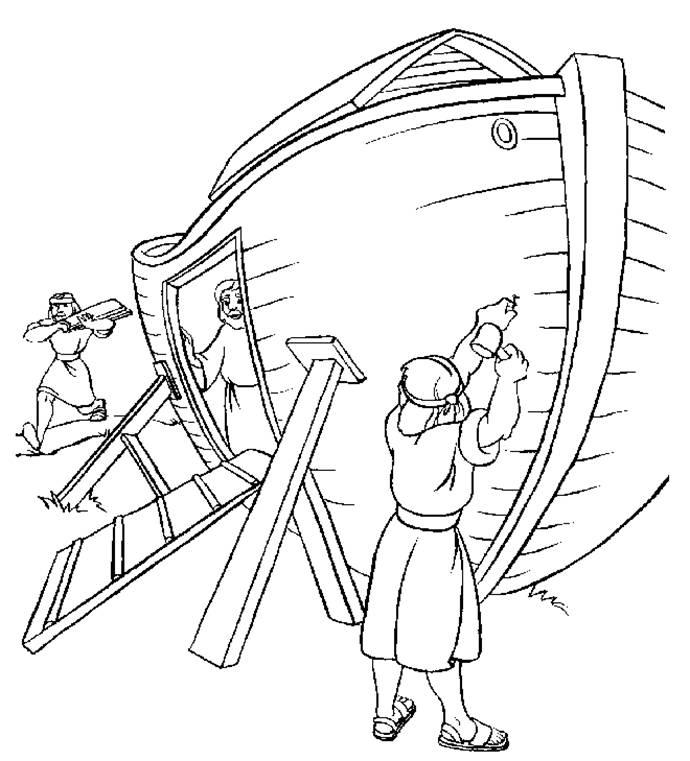# Dr. Pat's Orthodox Super Sunday School Curriculum

## Noah

NOAH

• Visit the Zoo again; see all the animals. Remind the children that all these were on the ark.
• Look around for pictures of rainbows – they’re everywhere! What do they say?
• Is there a storm this week? What was the storm like for Noah?
• Puzzle and coloring sheet.
• Read the story at bedtime.

Prayer: Lord, give us the faith of Noah no matter how bad things look.

# What did God say to Noah?

`g b e l e f r u i t f u l a n `
`d i m u i l l t i p l y a n d `
`f e r t i o t l l t h e e a r `
`t p a a h h n r s j a n h d d `
`p o k a f b h q u y r t b d d `
`g l g o w f u o i t b m u e p `
`j e g s q i e t y u e o y l b `
`d t s f r e n y e x z o t e u `
`m n c r s a y e k t o r r a p `
`j a e r h e h k n r a v e n l `
`s l o p x w v n o t i g e r c `
`d h e b t f j o m t v c c b o `
`w l e g x x v d d i p c f j w `
`e p z e a x c h u i t e f u s `
`y k x n p h g s g a a o t j c `

These animals were in the ark:

`antelope`
`cow`
`donkey`
`dove`
`elephant`
`giraffe`
`goat`
`horse`
`lion`
`monkey`
`parrot`
`pig`
`raven`
`sheep`
`squirrel`
`tiger`
`turtle`
`zebra`
` `
`__ __   __ __ __ __ __ __ __ __   __ __ __   __ __ __ __ __ __ __ __   `
` `
`__ __ __   __ __ __ __   __ __ __   __ __ __ __ __ . `

Coloring Pages:Building the ArkNoah welcomes the AnimalsThe Rainbow of God’s Promise